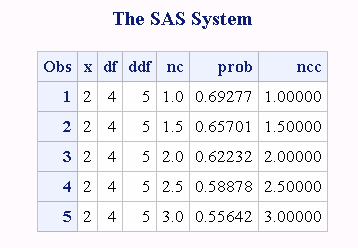# FNONCT Function

Returns the value of the noncentrality parameter of an F distribution.

 Category: Mathematical

## Syntax

FNONCT(x,ndf,ddf,prob)

### Required Arguments

#### x

is a numeric random variable.

 Range x ≥ 0

#### ndf

is a numeric numerator degree of freedom parameter.

 Range ndf > 0

#### ddf

is a numeric denominator degree of freedom parameter.

 Range ddf > 0

#### prob

is a probability.

 Range 0 < prob < 1

## Details

The FNONCT function returns the nonnegative noncentrality parameter from a noncentral F distribution whose parameters are x, ndf, ddf, and nc. If prob is greater than the probability from the central F distribution whose parameters are x, ndf, and ddf, a root to this problem does not exist. In this case a missing value is returned. A Newton-type algorithm is used to find a nonnegative root nc of the equation
$equation$
The following relationship applies to the preceding equation:
$equation$
In the equation, I (. . .) is the probability from the beta distribution that is given by the following equation:
$equation$
If the algorithm fails to converge to a fixed point, a missing value is returned.

## Example

```data work;
x=2;
df=4;
ddf=5;
do nc=1 to 3 by .5;
prob=probf(x,df,ddf,nc);
ncc=fnonct(x,df,ddf,prob);
output;
end;
run;
proc print;
run;```
FNONCT Example Output